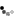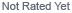Loading... Please wait...

If Statement

If Statement

The if() statement is the most basic of all programming control structures. It allows you to make something happen or not depending on whether a given condition is true or not. It looks like this:

if (someCondition) {
// do stuff if the condition is true
}

There is a common variation called if-else that looks like this:

if (someCondition) {
// do stuff if the condition is true
} else {
// do stuff if the condition is false
}

There's also the else-if, where you can check a second condition if the first is false:

if (someCondition) {
// do stuff if the condition is true
} else if (anotherCondition) {
// do stuff only if the first condition is false
// and the second condition is true
}

You'll use if statements all the time. The example below turns on an LED on pin 13 (the built-in LED on many Arduino boards) if the value read on an analog input goes above a certain threshold.

Hardware Required

• Arduino Board
• (1) Potentiometer or variable resistor
• (1) 220 ohm resistor
• (1) LED
• hook-up wire

Circuit

click the image to enlarge

image developed using Fritzing. For more circuit examples, see the Fritzing project page

Schematic:

click the image to enlarge

Code

In the code below, a variable called `analogValue` is used to store the data collected from a potentiometer connected to the Arduino on `analogPin 0`. This data is then compared to a threshold value. If the analog value is found to be above the set threshold the LED connected to digital pin 13 is turned on. If analogValue is found to be `<` threshold, the LED remains off.

/*
Conditionals - If statement

This example demonstrates the use of if() statements.
only if the LED goes above a certain threshold level. It prints the analog value
regardless of the level.

The circuit:
* potentiometer connected to analog pin 0.
Center pin of the potentiometer goes to the analog pin.
side pins of the potentiometer go to +5V and ground
* LED connected from digital pin 13 to ground

* Note: On most Arduino boards, there is already an LED on the board
connected to pin 13, so you don't need any extra components for this example.

created 17 Jan 2009
modified 9 Apr 2012
by Tom Igoe

This example code is in the public domain.

http://arduino.cc/en/Tutorial/IfStatement

*/

// These constants won't change:
const int analogPin = A0;    // pin that the sensor is attached to
const int ledPin = 13;       // pin that the LED is attached to
const int threshold = 400;   // an arbitrary threshold level that's in the range of the analog input

void setup() {
// initialize the LED pin as an output:
pinMode(ledPin, OUTPUT);
// initialize serial communications:
Serial.begin(9600);
}

void loop() {
// read the value of the potentiometer:

// if the analog value is high enough, turn on the LED:
if (analogValue > threshold) {
digitalWrite(ledPin, HIGH);
}
else {
digitalWrite(ledPin,LOW);
}

// print the analog value:
Serial.println(analogValue);
delay(1);        // delay in between reads for stability
}

• if()
• if...else
• digitalWrite()
• serial.begin()
• serial.print()
• For Loop - Control multiple LEDs with a For Loop.
• While Loop - Use a While Loop to calibrate a sensor while a button is being pressed.
• Switch Case - Choose between a number of discrete values in a manner that is the equivalent of using multiples If statements. This example shows how to divide a sensor's range into a set of four bands and to take four different actions depending on which band the result is in.
• Array: a variation on the For Loop example that demonstrates how to use an array.

New Products

•••••What's News

• Using a Generic STM32 board with Arduino

Using a Generic STM32 Board with ArduinoIf you haven't got one already, you can …

• Using a SIM800l GSM/GPRS Cellphone Module to send an SMS message

This example shows how to use a low-cost SIM800l GSM/GPS Module to send an SMS …

• Using MQTT with ESP8266

This blog entry is quick outline of using MQTT with a message broker to …